<...Previous...> <...Next...> Page 1 2 3 4 5 6 Math Home Ideas Home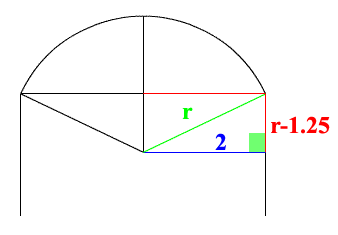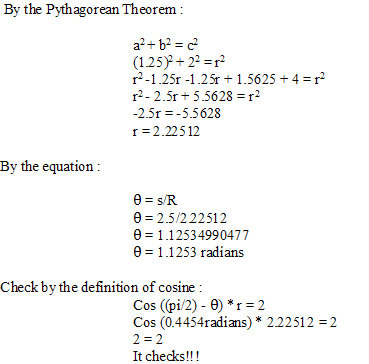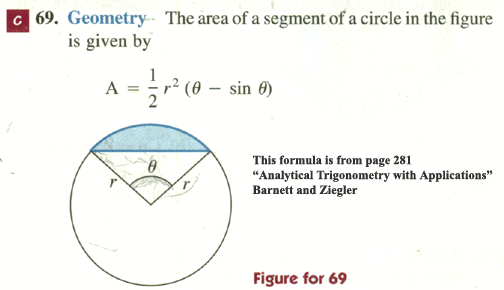This is the formula used to determine the area of the equation. Follow the next steps.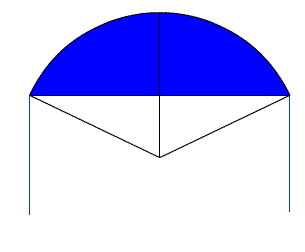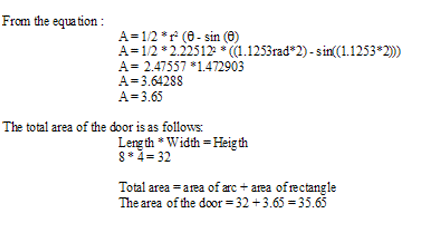Is the problem know totally solved using basic mathmatics??? There is a lot to learn from this problem. The steps to this problem could possibly be used to solve other problems. But the thing to study here is the relationship on how a change in the cosine or a change in the sine form a triangle. This problem has other solutions. But higher mathematics must be used to solve them. Remember if your have any questions or comments just leave them on the message board.

 <...Previous...> <...Next...> Page 1 2 3 4 5 6 Math Home Ideas Home